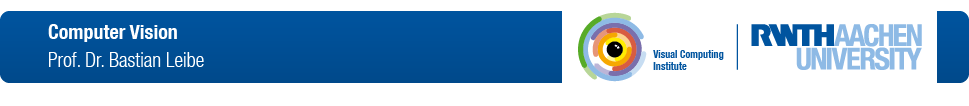# Machine Learning

SS 2016

Lecture

### Credits:

V3 + Ü1 (6 ECTS credits)
Find a list of current courses on the Teaching page.
Course Dates:

### Room

Lecture Mon, 16:15-17:45 AH IV
Lecture/Exercise Tue, 16:15-17:45 AH II

## Lecture Description

The goal of Machine Learning is to develop techniques that enable a machine to "learn" how to perform certain tasks from experience.

The important part here is the learning from experience. That is, we do not try to encode the knowledge ourselves, but the machine should learn it itself from training data. The tools for this are statistical learning and probabilistic inference techniques. Such techniques are used in many real-world applications. This lecture will teach the fundamental machine learning know-how that underlies such capabilities. In addition, we show current research developments and how they are applied to solve real-world tasks.

Example questions that could be addressed with the techniques from the lecture include

• Is this email important or spam?
• What is the likelihood that this credit card transaction is fraudulent?
• Does this image contain a face?

## Exercises

The class is accompanied by exercises that will allow you to collect hands-on experience with the algorithms introduced in the lecture.

There will be both pen&paper exercises and practical programming exercises based on Matlab (roughly 1 exercise sheet every 2 weeks). Please submit your hand written solutions of the exercises the day before the exercise class in the submission box at room 129, UMIC. Please submit your source code solutions through the L2P system.

We ask you to work in teams of 2-3 students.

## Literature

For most part, the lecture will follow the book by Bishop. Additional topics are covered in Duda & Hart's book Christopher M. Bishop, Pattern Recognition and Machine Learning, Springer, 2006 R.O. Duda, P.E. Hart, D.G. Stork, Pattern Classification, 2nd Edition, Wiley-Interscience, 2000 Wherever research papers are necessary for a deeper understanding, we will make them available on this web page.

## Matlab Resources

Course Schedule
Date Title Content Material
Introduction Introduction, Probability Theory, Bayes Decision Theory, Minimizing Expected Loss
Exercise 0 Intro Matlab
Prob. Density Estimation I Parametric Methods, Gaussian Distribution, Maximum Likelihood
Prob. Density Estimation II Bayesian Learning, Nonparametric Methods, Histograms, Kernel Density Estimation
Prob. Density Estimation III Mixture of Gaussians, k-Means Clustering, EM-Clustering, EM Algorithm
Linear Discriminant Functions I Linear Discriminant Functions, Least-squares Classification, Generalized Linear Models
Linear Discriminant Functions II Fisher Linear Discriminants, Logistic Regression, Iteratively Reweighted Least Squares
Exercise 1 Probability Density, GMM, EM
- no class (excursion week)
- no class (excursion week)
Statistical Learning Theory Statistical Learning Theory, VC Dimension, Structural Risk Minimization
Linear SVMs Linear SVMs, Soft-margin classifiers, nonlinear basis functions
Non-Linear SVMs Kernel trick, Mercer's condition, Nonlinear SVMs, Support Vector Data Description
Exercise 2 Linear Classifiers, Fisher Linear Discriminants, VC Dimension
Ensemble Methods and Boosting Model Combination, Bagging, Boosting, AdaBoost, Sequential Additive Minimization
Randomized Trees Randomized Decision Trees, Random Forests, Extremely Randomized Trees, Ferns
Deep Learning I Perceptrons, Multi-Layer Neural Networks, Learning with Hidden Units, Backpropagation
Deep Learning II Training Deep Networks, Minibatch Learning, Nonlinearities, Convergence of Gradient Descent, Momentum Method, RMSProp
Exercise 3 AdaBoost, Decision Trees, Random Forests
Graphical Models I Intro to Graphical Models, Bayesian Networks, Conditional Independence, Bayes Ball algorithm
Graphical Models II Markov Random Fields, Converting directed to undirected models
- no class (cancelled)
- no class (cancelled)
Graphical Models III Exact Inference, Message Passing, Belief Propagation, Factor Graphs, Sum-product algorithm
Applying MRFs & CRFs Junction Tree Algorithm, Loopy BP, Applications of MRFs, CRFs, Graph Cuts
Repetition Repetition
Exercise 4 Bayes Nets, Inference, Belief Propagation
Exam 1 Matriculation number < 323200
Exam 1 Matriculation number >= 323200 and < 362188
Exam 1 Matriculation number >= 362188
Exam 2 Matriculation number < 320000
Exam 2 Matriculation number >= 320000